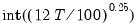buroot
Carries out unit root tests which allow for a single breakpoint.
Syntax
series_name.buroot(options)
Basic Specification Options
You should specify the exogenous variables and order of dependent variable differencing in the test equation using the following options:

 exog=arg (default=“const”) Specification of exogenous trend variables in the test equation: “const” “trend” (include a constant and a linear time trend). dif=integer (default=0) Order of differencing of the series prior to running the test. Valid values are {0, 1, 2}.
Break Options

 break=arg (default=“const”) Specification of breaking trend variables in the test equation: “const” (intercept only), “both” (intercept and trend), “trend” (trend only). The latter two are applicable only if “exog=trend”). breakmethod=arg (default=“dfuller”) Method of specifying the break date: “dfuller” (minimize Dickey-Fuller t-statistic), “minincpt” (minimize intercept break t-statistic), “maxincpt” (maximize intercept break t-statistic), “absincpt” (maximize intercept break absolute t-statistic), “mintrend” (minimize trend break t-statistic), “maxtrend” (maximize trend break t-statistic), “abstrend” (maximize trend break absolute t-statistic), “both” (maximize joint intercept and trend break F-statistic), “user” (fixed break date specified using the “userbreak=” option). trim=arg (default=10) Trimming percentage for allowable break dates to consider in automatic break selection (applicable if the specified break method selects a date on the basis of intercept or trend break coefficients). userbreak=dateobs User-specified break date. type=arg (default="io") Break type: innovation outlier (“io”), additive outlier (“ao”).
Lag Difference Options
Specifies the number of lag difference terms to be included in the test equation. The default is to perform automatic selection using the Schwarz information criterion. You may specify a fixed lag using the “lag=” option.

 lagmethod=arg (default=“sic”) Method for selecting lag length (number of first difference terms) to be included in the Dickey-Fuller test regressions:“aic” (Akaike), “sic” (Schwarz), “hqc” (Hannan-Quinn), “msaic” (Modified Akaike), “msic” (Modified Schwarz), “mhqc” (Modified Hannan-Quinn), “tstat” (Ng-Perron first backward significant t-statistic), “fstat” (significant F-statistic). lag=integer Use-specified fixed lag. maxlag=integer Maximum lag length to consider when performing automatic lag length selection.default=lagpval=arg (default=0.1) Probability value for test-based automatic lag selection (when “lagmethod = tstat” and “lagmethod=fstat).
General options

 nograph Do not display breakpoint selection graph (by default, EViews shows a graph of all of the individual unit root tests and AR coefficients when there is endogenous breakpoint selection). output=arg Output matrix containing individual unit root regression results for all candidate break dates. Each row contains the relevant workfile observation ID (as reported by @TREND), AR coefficient, AR coefficient standard error, number of observations, number of coefficients, number of lags, and if applicable, the t-statistic or F-statistic used in break selection. prompt Force the dialog to appear from within a program. p Print output from the test.
Cross-references
See “Unit Root Tests with a Breakpoint” for additional discussion.